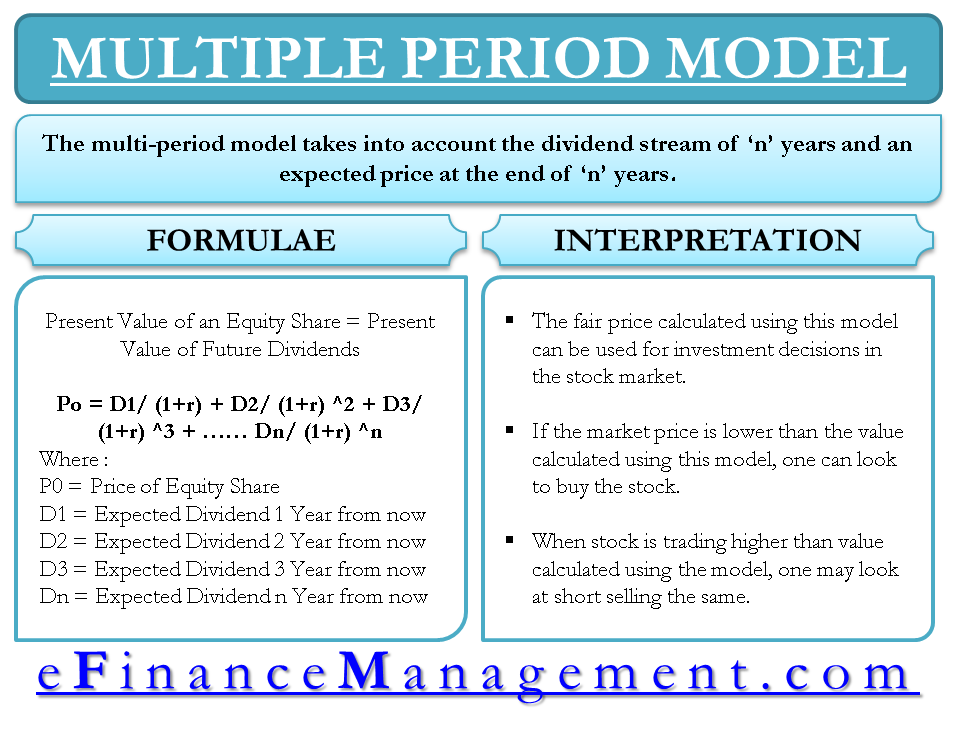# Multiple Period Model of Equity Valuation – Dividend Discount Model

Multiple Period Model of Equity Valuation is also a dividend discount model. Like any other discounted cash flow model aims at arriving at the intrinsic/fair value of the stock. The multi-period model takes into account the dividend stream of ‘n’ years and an expected price at the end of ‘n’ years. It essentially discounts both dividends and expected price using an appropriate discount rate to arrive at the fair value. This model is appropriate for investors having a holding period in mind. The multi-period model will take those many years into account to arrive at the intrinsic value of the stock.

## Brief on Equity Share as an Investment

Equity share is an investment alternative, whereby an investor can take part in a company’s capital and share the profits of the company. They also have a claim on assets of the company but they never get their principal back like debt investments. In the case of listed companies, an investor can sell his stake in the market.## How to Calculate Stock Price Using Multi-Period Dividend Discount Model Formula?

In essence, the price can be calculated as follows:

### Formula for Multi-Period Model

For valuing anything using a discounting/present value formula, the first thing that is required is the Cash Inflows. In this case, the cash inflow is the ‘Expected Dividend’ ought to be received every year. In simple terms, it can be stated as below:

Present Value of an Equity Share = Present Value of Future Dividends

Po = D1/ (1+r) + D2/ (1+r) ^2 + D3/ (1+r) ^3 + …… Dn/ (1+r) ^n

Where,

Po = Price of the equity share

D1 = expected dividend 1 year from now

D2 = expected dividend 2 years from now

Dn = expected dividend n years from now.

r = expected rate of return (cost of equity)

This formula takes into account an infinite number of years, which is difficult to forecast in a real-world situation, and one may use perpetuity as a dividend value after a few years beyond which forecast is difficult. For shorter holding periods, one can use the multi-period to value the stock price as listed in the below example.

### Example of Multi-Period Model

Let us look at an example where a particular investor with a 5-year horizon wants to calculate the fair value of the stock.  Given the expected dividend stream for the next 5 years and expected price after 5 years, one can arrive at the intrinsic value of the stock using an appropriate discount rate. The following information is available:

D1 = \$ 2, D2 = \$ 3, D3 = \$ 4, D4 = \$ 5, D5 = \$ 6

Expected stock price after 5 yrs = \$ 120

Cost of equity (required rate of return) = 10%

Using the information available, the value of the stock can be calculated as follows:

 Tenor Cash Flow Discount Rate Present Value 1 \$ 2 10% 2 / (1+10%)^1 = 1.82 2 \$ 3 10% 3 / (1+10%)^2 = 2.48 3 \$ 4 10% 4 / (1+10%)^3 = 3.00 4 \$ 5 10% 5 / (1+10%)^4 = 3.42 5 \$ 6 10% 6 / (1+10%)^5 = 3.72 5 \$ 120 10% 120 / (1+10%)^5 = 74.51

The intrinsic value of the stock will be a summation of all present values and in our case, this sums up to \$ 88.95.

## Interpretation of Multi-Period Model

The fair price arrived using the multi-period model can be used by investors to make investment decisions in the stock market. If the market price is lower than the value calculated using this model, one can look to buy the stock as the stock is trading undervalued and vice versa when a stock is trading higher than the value calculated using the model, one may look at short selling the same. However, if the market prices seem fair one may need to revisit the estimates of the stock price at the end of the holding period, especially the dividend estimations and the cost of equity.  To estimate intrinsic value closer to reality one needs to assume infinite periods and hence one may look at the other multi-period dividend discount models like two-stage growth model, H model or three-stage growth model which will help the investor to reduce the error in the estimation of fair/intrinsic value.1,2

1.
sapm. psnacet. January 2019. [PDF]
2.
Financial Resources. educ. January 2019. [PDF]
Last updated on : August 14th, 2019